Next: Non-standard uses of signatures Up: Modular mappings Previous: Primes suitable for nested

## Computing signatures of logarithms and inverse trigonometrics

Computing signatures of logarithms is more complicated in the sense that we do not know how to compute them in time polynomial in the size of the problem. The signatures will be computed as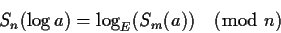where n and m = kn+1 are primes, E=Sm(e)=pk is the base of the exponential function and p is a primitive root (mod m). The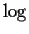is the discrete logarithm, sometimes called the index function. It is defined by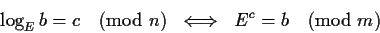Notice the different ground fields on which we are computing here. This is similar to thefunction, where the exponent arithmetic was done on a different field, but in reverse.

If the signature of the argument is 0, we cannot compute the discrete logarithm. This is a case analogous to a division by zero, and we will proceed as with it. I.e. we will recursively test whether the argument is 0. If it is, then the computation does not make sense and it is aborted. If it is not, then we have found an n for which the signature is non-zero, and we continue the computation in this field.

With such a value for E, not being a primitive root, there will be discrete logarithms that will not be possible to compute. This problem is tricky to solve, but we can use the fact that the range of the discrete logarithm is normally 0 ... m-2, and we fold this range to 0 ... n-1. Let r=pn, then r is a kth root of unity, and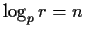. Multiplying the arguments of the logarithms by any power of rwill not change their signature. If the base of the logarithms is p, then it is clear thatIn other words, the signatures of logarithms group values of the arguments in equivalence classes of size k. The generator of these classes of equivalence is r. When we compute discrete logarithms base E, then this class of equivalence will have one member having a logarithm, and all the others being not representable. We will use as a signature of any argument, the signature of any of the members of its equivalence class. It turns out that this is equivalent to computing the discrete logarithms as: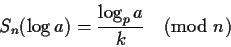A numerical example helps to understands all these computations and problems. Assume that n=13 and m=53 and hence k=4. Let p=2 which is a primitive root (mod 53). Then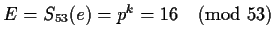and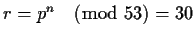. We would like to prove that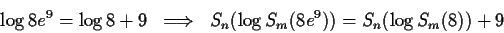The signatures of the arguments are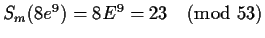and Sm(8)=8. Now,does not exist. I.e. there is no value c such that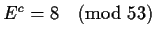. But 8 is in a class of equivalence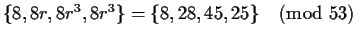. Of the four components of this equivalence class, only 28 has a discrete logarithm base E,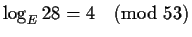. Similarly for 23,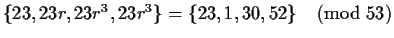, and only 1 has a logarithm,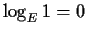. Notice that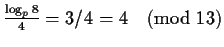and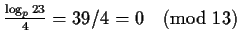. This gives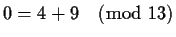which verifies the initial equation.Next: Non-standard uses of signatures Up: Modular mappings Previous: Primes suitable for nested
Gaston Gonnet
1999-07-04